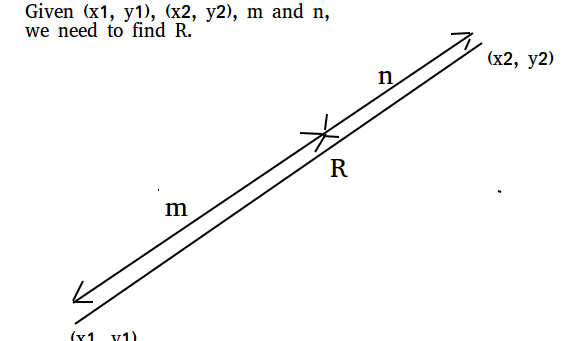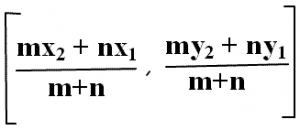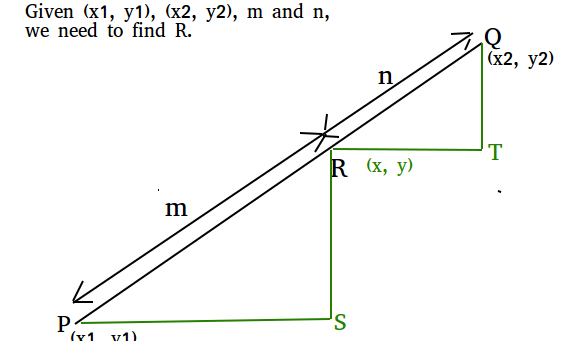Related Articles

# Section formula (Point that divides a line in given ratio)

• Difficulty Level : Easy
• Last Updated : 13 Apr, 2021

Given two coordinates (x1, y1) and (x2, y2), and m and n, find the co-ordinates that divides that divides the line joining (x1, y1) and (x2, y2) in the ratio m : nExamples:

```Input : x1 = 1, y1 = 0, x2 = 2 y2 = 5,
m = 1, n = 1
Output : (1.5, 2.5)
Explanation: co-ordinates (1.5, 2.5)
divides the line in ratio 1 : 1

Input : x1 = 2, y1 = 4, x2 = 4, y2 = 6,
m = 2, n = 3
Output : (2.8, 4.8)
Explanation: (2.8, 4.8) divides the line
in the ratio 2:3```

The section formula tells us the coordinates of the point which divides a given line segment into two parts such that their lengths are in the ratio m : n## C++

 `// CPP program to find point that divides``// given line in given ratio.``#include ``using` `namespace` `std;` `// Function to find the section of the line``void` `section(``double` `x1, ``double` `x2, ``double` `y1,``              ``double` `y2, ``double` `m, ``double` `n)``{``    ``// Applying section formula``    ``double` `x = ((n * x1) + (m * x2)) /``                            ``(m + n);``    ``double` `y = ((n * y1) + (m * y2)) /``                             ``(m + n);` `    ``// Printing result``    ``cout << ``"("` `<< x << ``", "``;``    ``cout << y << ``")"` `<< endl;``}` `// Driver code``int` `main()``{``    ``double` `x1 = 2, x2 = 4, y1 = 4,``           ``y2 = 6, m = 2, n = 3;``    ``section(x1, x2, y1, y2, m, n);``    ``return` `0;``}`

## Java

 `// Java program to find point that divides``// given line in given ratio.``import` `java.io.*;` `class` `sections {``    ``static` `void` `section(``double` `x1, ``double` `x2,``                        ``double` `y1, ``double` `y2,``                        ``double` `m, ``double` `n)``    ``{``        ``// Applying section formula``        ``double` `x = ((n * x1) + (m * x2)) /``                    ``(m + n);``        ``double` `y = ((n * y1) + (m * y2)) /``                    ``(m + n);`  `        ``// Printing result``        ``System.out.println(``"("` `+ x + ``", "` `+ y + ``")"``);``    ``}` `    ``public` `static` `void` `main(String[] args)``    ``{``        ``double` `x1 = ``2``, x2 = ``4``, y1 = ``4``,``               ``y2 = ``6``, m = ``2``, n = ``3``;``        ``section(x1, x2, y1, y2, m, n);``    ``}``}`

## Python

 `# Python program to find point that divides``# given line in given ratio.``def` `section(x1, x2, y1, y2, m, n):` `    ``# Applying section formula``    ``x ``=` `(``float``)((n ``*` `x1)``+``(m ``*` `x2))``/``(m ``+` `n)``    ``y ``=` `(``float``)((n ``*` `y1)``+``(m ``*` `y2))``/``(m ``+` `n)` `    ``# Printing result``    ``print` `(x, y)` `x1 ``=` `2``x2 ``=` `4``y1 ``=` `4``y2 ``=` `6``m ``=` `2``n ``=` `3``section(x1, x2, y1, y2, m, n)`

## C#

 `// C# program to find point that divides``// given line in given ratio.``using` `System;` `class` `GFG {``    ` `    ``static` `void` `section(``double` `x1, ``double` `x2,``                        ``double` `y1, ``double` `y2,``                          ``double` `m, ``double` `n)``    ``{``        ` `        ``// Applying section formula``        ``double` `x = ((n * x1) + (m * x2)) /``                                    ``(m + n);``                                    ` `        ``double` `y = ((n * y1) + (m * y2)) /``                                   ``(m + n);` `        ``// Printing result``        ``Console.WriteLine(``"("` `+ x + ``", "` `+ y + ``")"``);``    ``}` `    ``// Driver code``    ``public` `static` `void` `Main()``    ``{``        ` `        ``double` `x1 = 2, x2 = 4, y1 = 4,``                ``y2 = 6, m = 2, n = 3;``                ` `        ``section(x1, x2, y1, y2, m, n);``    ``}``}` `// This code is contributed by vt_m.`

## PHP

 ``

## Javascript

 ``

Output:

`(2.8, 4.8)`

How does this work?```From our diagram, we can see,
PS = x – x1 and RT = x2 – x

We are given,

PR/QR = m/n

Using similarity, we can write
RS/QT = PS/RT = PR/QR

Therefore, we can write
PS/RR = m/n
(x - x1) / (x2 - x) = m/n

From above, we get
x = (mx2 + nx1) / (m + n)

Similarly, we can solve for y.```

Attention reader! Don’t stop learning now. Get hold of all the important mathematical concepts for competitive programming with the Essential Maths for CP Course at a student-friendly price. To complete your preparation from learning a language to DS Algo and many more,  please refer Complete Interview Preparation Course.

My Personal Notes arrow_drop_up